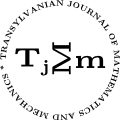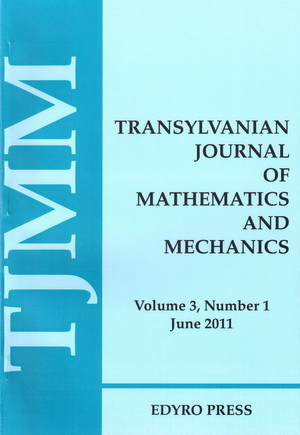ISSN: 2067-239X
ISSN(on-line): 2067-239X

Indexed in:
Mathematical Reviews
Zentralblatt MATH
EBSCO

Front cover# Volume 3 (2011), Number 1

## On a Certain Subclass of Analytic Functions Involving Differential Operators

### Author(s): AFAF ABUBAKER AND MASLINA DARUS

Abstract: In this paper we introduce a new subclass of normalized analytic functions in the open unit disc which is defined by a certain differential operator. A coefficient inequality, distortion Theorems and extreme points of differential operator for this class are given. We also discuss the boundedness properties associated with partial sums of functions in the class $T{S}_{\lambda ,\delta }^{\sigma ,s}\left(\beta ,\gamma ,n\right)$.

## A Companion of Ostrowski's Inequality with Applications

Abstract: An inequality for a companion of Ostrowski's integral inequality is proved. Applications to a composite quadrature rule and to probability density functions are considered.

## Some Differential Equations of First Order with Mixed Modified Arguments and with a Parameter

### Author(s): MARIA DOBRIȚOIU

Abstract: In this paper we study the solution of a generalization of a type of differential equation with mixed modified argument and with a parameter. Using an observation from V.A. Ilea  we present two result regarding the existence and uniqueness and the data dependence of the solution of this differential equation. An example is also given here.

## Growth and Weighted Polynomial Approximation of Analytic Functions

### Author(s): DEVENDRA KUMAR

Abstract: Let ${H}_{R}$ be the class of functions analytic in ${G}_{R}$ but not in ${G}_{{R}^{\prime }}$ if $R<{R}^{\prime }$, ${G}_{{R}_{o}}=\text{int}\text{\hspace{0.17em}}{S}_{{R}_{o}}$, $0<{R}_{o} and ${S}_{{R}_{o}}=\left\{z\in ℂ:|z{e}^{1-z}|={R}_{o},\text{\hspace{0.17em}}|z|\le 1\right\}$. This paper deals with the characterization of rate of decay of weighted approximation error on ${S}_{{R}_{o}}$, in terms of order and type of $f\in {H}_{R}$.

## A Generalized Goldbach (Descartes) Binary Problem

### Author(s): A. PERETTI AND M. BAICA

Abstract: In this paper the authors will introduce a Diophantine Equation which can be considered as the generalized Descartes (misnamed Goldbach) binary problem. The authors do not solve the problem but give some important hints which could lead to the solution of this problem. Also we should not be surprised if it will take again more than 360 years until a complete solution will be given to our generalized conjecture.

## Certain Subclass of Analytic Functions Associated with Caputo's Fractional Differentiation

### Author(s): JAMAL SALAH AND MASLINA DARUS

Abstract: In this paper, we define a class ${T}_{\eta ,\lambda }\left(\alpha ,\beta ,A,B\right)$ of analytic functions involving the integral operator given by the authors. Several results like characterization property, distortion theorem and other interesting properties of the same class are provided.

## On Uniform Exponential Stability of Variational Difference Equations in Banach Spaces

### Author(s): MIHAELA AURELIA TOMESCU

Abstract: In this paper we study the uniform exponential stability property for variational difference equations in Banach spaces. Characterizations of this concept are given. The obtained results can be considered as variants of the classical results due to E.Barbashin () and R.Datko () for variational difference equations.